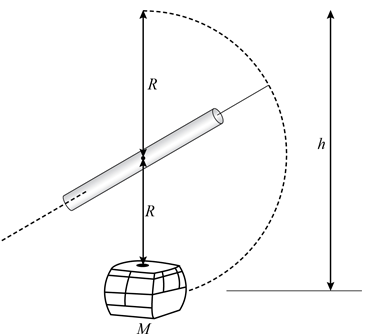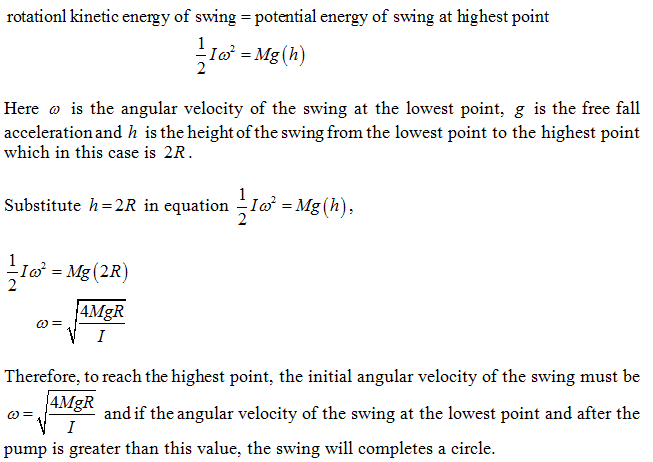×#### Thank you for registering.

One of our academic counsellors will contact you within 1 working day.

Click to Chat

1800-1023-196

+91-120-4616500

CART 0

• 0

MY CART (5)

Use Coupon: CART20 and get 20% off on all online Study Material

ITEM
DETAILS
MRP
DISCOUNT
FINAL PRICE
Total Price: Rs.

There are no items in this cart.
Continue Shopping
```
Can you "pump" a swing so that it turns in a complete circle, moving completely around its support? Assume (if you wish) that the seat of the swing is connected to its support by a rigid rod rather than a rope or chain. Explain your answer.

```
5 years ago

```							When one pumps a swing, the increase in angular velocity of the swing is due to the decrease in rotational inertia of the person on the swing. But when the swing moves upwards, the gravitational force exerts a torque on the swing and rotates the swing in the opposite direction. This angular velocity from this torque when becomes greater than the angular velocity due to the decrease in rotational inertia, the swing moves back to its equilibrium position.Therefore if the swing has sufficient energy during the pump, that the increased angular velocity is more than the angular velocity given by the torque due to gravitational force, the swing will complete a circle.Mathematically, one can derive the angular velocity that the swing must have after the pump so that the swing can completes a circle as:Assume that the rotational inertia of the swing and person about the axis of rotation is I, R is the length of the rigid rod and M is the combined mass of the swing and the person on swing (refer figure below).From the conservation of energy, one has```
5 years ago
```							When one pumps a swing, the increase in angular velocity of the swing is due to the decrease in rotational inertia of the person on the swing. But when the swing moves upwards, the gravitational force exerts a torque on the swing and rotates the swing in the opposite direction. This angular velocity from this torque when becomes greater than the angular velocity due to the decrease in rotational inertia, the swing moves back to its equilibrium position.Therefore if the swing has sufficient energy during the pump, that the increased angular velocity is more than the angular velocity given by the torque due to gravitational force, the swing will complete a circle.Mathematically, one can derive the angular velocity that the swing must have after the pump so that the swing can completes a circle as:Assume that the rotational inertia of the swing and person about the axis of rotation is I,R is the length of the rigid rod and M is the combined mass of the swing and the person on swing (refer figure below).From the conservation of energy, one has```
5 years ago
Think You Can Provide A Better Answer ?

## Other Related Questions on Mechanics

View all Questions »### Course Features

• 101 Video Lectures
• Revision Notes
• Previous Year Papers
• Mind Map
• Study Planner
• NCERT Solutions
• Discussion Forum
• Test paper with Video Solution### Course Features

• 110 Video Lectures
• Revision Notes
• Test paper with Video Solution
• Mind Map
• Study Planner
• NCERT Solutions
• Discussion Forum
• Previous Year Exam Questions